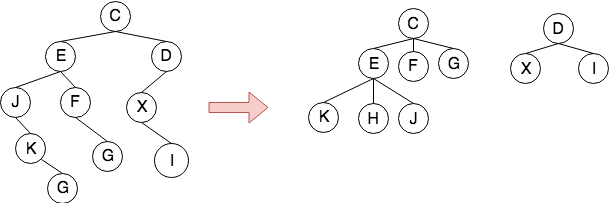# 树与森林

### 树与线性结构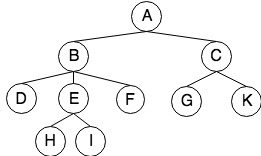### 树的特性

• 使用集合来描述
• 树(tree)是包括n个节点的有限集合T(n≥1)，它有一个特定的节点，称为根，除了根之外的节点被划分成$m$个不想交的集合，$T_1,T_2,…,T_m$，每个集合又都是树，称为$T$的子树
• 树也可以用集合表示，例如上面的树，用集合表示为:
• 节点结合 K={ A,B,C,D,E,F,G,H,I,J }
• $K$上的关系 r= { <A,B>,<A,C>,<B,D>,<B,E>,<B,F>,<C,G>,<C,H>,<E,I>,<E,J>}
• 树是有序有向的
• 树的兄弟节点之间是有序的，对于子树来说，和它平行的子树称为兄弟（sibling），兄弟之间亦有次序，可以从最左子树开始编号，向右递增
• 此外，度为2的有序树并不是二叉树。因为第一子节点被删除后,第二子节点自然顶替成为第一
• 连通无环图
• 从图论的角度讲，树是一种在连通和无环之间达到平衡的一种图。在保证连通的前提下，由于树不成环，它的边数能达到最少，因此它是一种极小的连通图；但是由于没有环路，树又可以使用最多的边，因此，它又是一种极大的无环图
• 在有根节点的前提下，任一节点$v$与根节点之间存在唯一路径 $path(v,r) = path(v)$，即每个节点可用到达该节点的边数来表示，我们称这个值为$c$。如果同一类的节点具有相同的$c$值，我们称这些节点为等价类，例如上图中深度为2的等价对有$<D,E>,<E,F>,<F,G>,<G,K>$
• 树的深度和层次
• 根节点是所有节点的公共祖先，深度为0
• 没有后代的节点称为叶子节点，树中必然存在叶子节点
• 所有叶子节点中深度最大者称为树的高度 $height(v) = height(subtree(v))$
• 树的规模
• 令一棵树中的节点数为$n$，对每个节点 $r$ 的度数为$degree(r)$, 边的数目为$e$，他们之间有如下关系:

这个公式可以表明$n$个节点的树有 $n-1$条边，树的边数和节点数是同阶的，一棵树的规模如果可以度量为节点数+边数 $(n+e)$，那么从渐进的角度来看，树的规模也是和节点数或者边数是同阶的，因此后面讨论时间复杂度时可以以树节点的数目为参照

• 森林
• 零棵或者多棵不相交的树的集合
• 一棵树，删掉树根，其子树就构成了森林
• 加入一个节点作为根，森林就转化成了一棵树

### 森林和二叉树

• 森林转二叉树

1. $B(F)$的根是$T_1$的根
2. $B(F)$的左子树为$T_1$除去根节点后的子树组成的二叉树3
3. $B(F)$的右子树为${T_2,T_3}$组成的二叉树。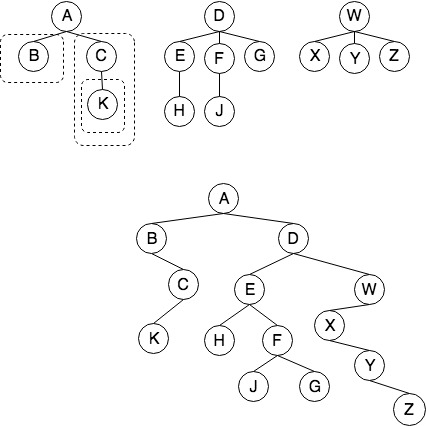1. 在森林中的所有兄弟节点之间加一连线
2. 对每个节点，去掉除了与第一个孩子之外的其他所有连线
3. 调整位置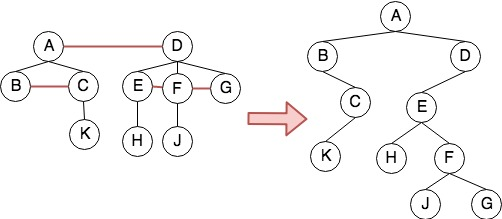• 二叉树转森林

1. 若$B$为空，则$F(B)$是空的森林
2. 若$B$不为空，则$F(B)$是一棵树$T_1$加上森林$F(B_R)$其中树$T_1$ 的根为root，root的子树为$F(B_L )$

1. 将除根节点以外的右子节点都与根节点相连
2. 去掉所有父节点和其右子节点的连线
3. 调整位置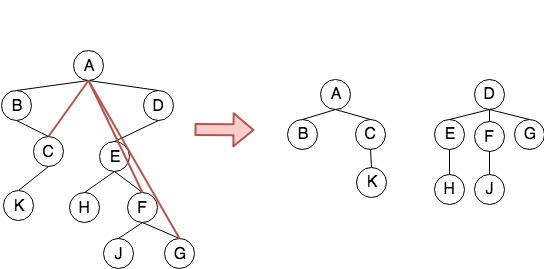### 树的Interface

template<class T>
class Tree {
public:
Tree(); // 构造函数
virtual ~Tree(); // 析构函数

void CreateRoot(const T& rootValue); // 创建值为rootValue的根节点
bool isEmpty(); // 判断是否为空树

//
TreeNode<T>* getRoot(); // 返回树中的根节点
TreeNode<T>* Parent(TreeNode<T> *current); // 返回父节点
TreeNode<T>* PrevSibling(TreeNode<T> *current); //返回前一个兄弟

//修改操作
void DeleteSubTree(TreeNode<T> *subroot); // 删除以subroot子树
void Insert

//遍历
void RootFirstTraverse(TreeNode<T> *root); // 先根深度优先遍历树
void RootLastTraverse(TreeNode<T> *root); // 后根深度优先遍历树
void WidthTraverse(TreeNode<T> *root); // 广度优先遍历树
};


## 树的几种实现方式

• 父节点表示法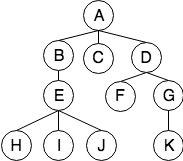index:      0 1 2 3 4 5 6 7 8 9 10
data:       A B C D E F G H I J K
parent:    -1 0 0 0 1 3 3 4 4 4 6


template<class T>
T data;
int parent;
};
template<class T>
class Tree {
vector<TreeNode> nodes; //数组
int n; //节点数
Tree
}


1. 空间复杂度：$O(n)$
2. 时间复杂度：
• 查找parent()节点: $O(1)$
• 查找root()节点: $O(n)$或$O(1)$
• 查找leftMostChild()节点：$O(n)$
• 查找sibling()节点：$O(n)$
• 动态数组表示法

index:      0     1  2  3       4     5 6 7 8 9 10
data:       A     B  C  D       E     F G H I J K
parent:    -1     0  0  0       1     3 3 4 4 4 6
children:   |     |     |       |       |
[1,2,3 ]  [5,6]  [7,8,9]   

template<class T>
int parent;
T data;
vector<TreeNode* >children; //可以用数组，也可以用链表
};
//Rootfirst Traverse
void traverse(TreeNode* root){
visit(root);
for(auto child : root->children){
traverse(child);
}
}


1. 森林中只有以一棵树
2. 适合构造一颗静态的树，用于遍历或者搜索
3. 不适合动态插入或者删除节点
• 二叉链表示法

1. 森林中最左边的子树，记为T1，它的根节点为树T的根节点
2. T的左子节点为T1的最左子树，T的右子节点是T1的右侧兄弟节点T2
3. 对于T2，重复第2步，它的左节点为自己的最左子树，它的右节点为T2的右侧兄弟节点T3
4. T3, T4...以此类推

template<class T>
private:
T m_Value; // 树节点的值
TreeNode<T> *pChild; // 第一个左孩子指针
TreeNode<T> *pSibling; // 右兄弟指针
public:
TreeNode(const T& value); // 拷贝构造函数
virtual ~TreeNode() {}; // 析构函数
...
};


1. 采用宽搜
2. 令森林中所有子树的根节点入队
3. 访问队列，对于每个弹出的根节点，检查它的LeftMostChild,如果和待查找节点相同，则返回该根节点。不同则入栈该，指针指向其兄弟节点，即在该层上进行宽搜
4. 重复过程3，直到找到该节点
TreeNode<T>* Parent(TreeNode<T> *current){
queue<TreeNode<T>*> aQueue;
TreeNode<T>* pointer = root; //树的根节点
TreeNode<T>* father = upperLevelPointer =  NULL;
//森林中所有子树根节点进队列
if(current != NULL && pointer != current){
while(pointer != NULL){
if(current == pointer){
break;
}
aQueue.push(pointer); //入队
pointer = pointer->RightSibling(); //该树根节点的右兄弟节点
}
}
while(!aQueue.empty()){
pointer = aQueue.front();
aQueue.pop();
upperLevelPointer = pointer;//维护该层指针
pointer = pointer -> LeftMostChild(); //水平遍历下一层
while(pointer){
if(current == pointer){
father = upperLevelPointer ;
break;
}else{
aQueue.push(pointer);
pointer = pointer -> RightSibling();
}
}
}
return father;
}


## 树的遍历

• 深度优先遍历
1. 先根遍历，对应二叉树的前序遍历
2. 后根遍历，对应二叉树的中序遍历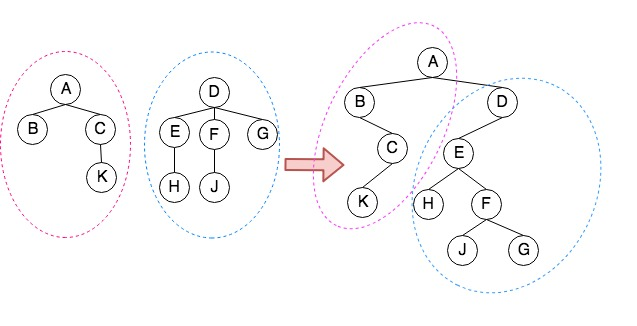//对于先根遍历，其思路是先根节点，然后左孩子树，然后右兄弟节点
void RootFirstTraverse(TreeNode<T>* root){
while(root != NULL){
//访问节点
visit(root);
// 遍历第1棵树根的子树森林(树根除外)
RootFirstTraverse(root->LeftMostChild);
RootFirstTraverse(root->RightSibling);
}
}
//后根遍历
RootLastTraverse(TreeNode<T> * root) {
while (root != NULL) {
// 遍历第一棵树根的子树森林
RootLastTraverse(root->LeftMostChild());
Visit(root->Value()); // 访问当前节点
root = root->RightSibling(); // 遍历其他树
}
}

• 宽度优先遍历森林
1. 首先依次访问层数为0的节点
2. 然后依次访问层数为1的节点
3. 直到访问完最下一层的所有节点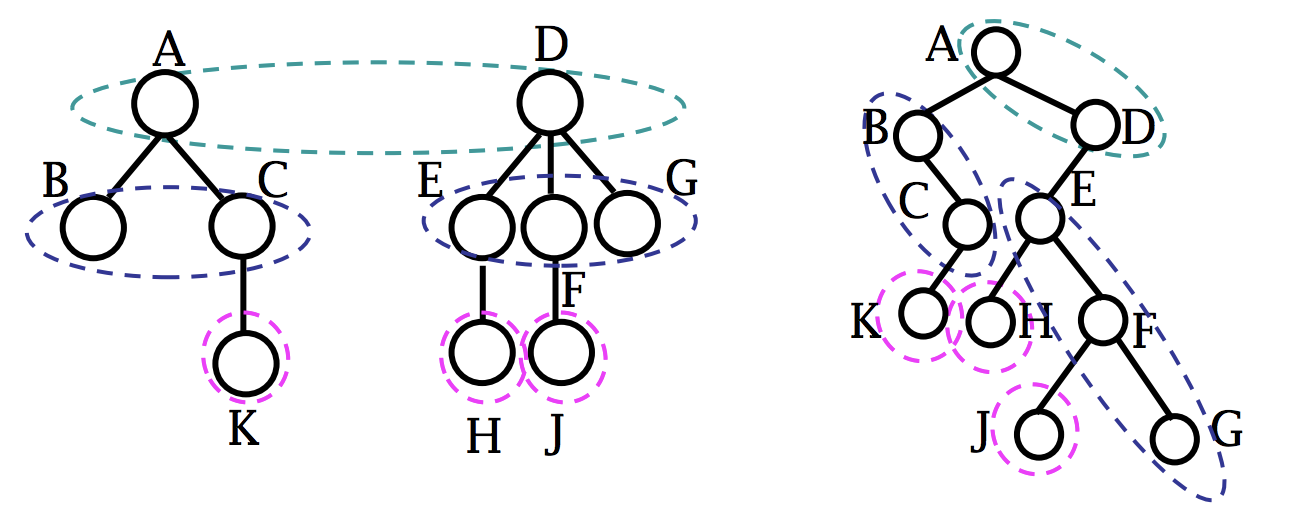void WidthTraverse(TreeNode<T> * root) {
queue<TreeNode<T>* > aQueue;
TreeNode<T> * pointer = root;
//森林中所有子树的根节点入队
while (pointer != NULL) {
aQueue.push(pointer); // 当前节点进入队列
pointer = pointer->RightSibling(); // pointer指向右兄弟
}
while(!aQueue.empty()){
pointer = aQueue.front();
aQueue.pop(); // 当前节点出队列
Visit(pointer->Value()); // 访问当前节点
pointer = pointer-> LeftMostChild(); // pointer指向最左孩子
while(pointer != NULL){ // 当前节点的子节点进队列
aQueue.push(pointer);
pointer = pointer->RightSibling();
}
}
}


## 树的序列化

-----------------------
| ltag | info | rtag |
-----------------------

info：结点的数据
rtag：二叉树结点没有右子结点,rtag为 1，否则为0
ltag：二叉树结点没有左子结点,ltag为 1，否则为0


rtag 0 0 1 1 1 0 1 0 1 1
info A B C K D E H F J G
ltag 0 1 0 1 0 0 1 0 1 1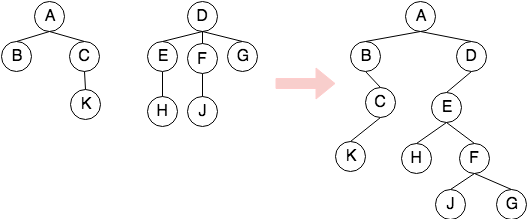rtag 0 0 0 0 1 0 1 1 0 1
info C E J K L F G D X I
ltag 0 0 1 1 1 1 1 0 1 1
`
1. 逐个读取存储节点，new一个新节点
2. C节点左右tag的值都为0，说明它有左右节点，但是还不知道是谁，先入栈保存，重复1
3. E节挂载到C的左节点，此时C的ltag为1。E和C一样，左右tag的值都为0，先入栈，重复1
4. J节点挂在到E的左节点，此时E的ltag为1。J的ltag为1，没有左孩子，但是右孩子为0，需要填补，因此入栈，重复1
5. K节点挂载到J的右结点，此时J的左右tag都为1，J出栈；同时K的rtag为0，需要填补，入栈，重复1。
6. L节点挂载到K的右结点，此时K的左右tag都为1，K出栈；同时L的左右tag也为1；不需入栈，重复1
7. 此时栈顶为E节点，将F节点挂载到E的右节点，此时E的左右tag都为1，E出栈；F的rtag为0,需要填补，入栈，重复1
8. G的操作和L类似，重复1
9. 此时栈中只剩C，因此D要挂在到C的右结点
10. D,X,I的恢复过程类似